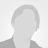# CCOMP: An efficient algorithm for complex roots computation of determinantal equations

Published: 6 Nov 2017 | Version 1 | DOI: 10.17632/x6fx4zssft.1
Contributor(s):
• Grigorios P. ZourosGrigorios P. Zouros
National Technical University of Athens

## Description of this data

In this paper a free Python algorithm, entitled CCOMP (Complex roots COMPutation), is developed for the efficient computation of complex roots of determinantal equations inside a prescribed complex domain. The key to the method presented is the efficient determination of the candidate points inside the domain which, in their close neighborhood, a complex root may lie. Once these points are detected, the algorithm proceeds to a two-dimensional minimization problem with respect to the minimum modulus eigenvalue of the system matrix. In the core of CCOMP exist three sub-algorithms whose tasks are the efficient estimation of the minimum modulus eigenvalues of the system matrix inside the prescribed domain, the efficient computation of candidate points which guarantee the existence of minima, and finally, the computation of minima via bound constrained minimization algorithms. Theoretical results and heuristics support the development and the performance of the algorithm, which is discussed in detail. CCOMP supports general complex matrices, and its efficiency, applicability and validity is demonstrated to a variety of microwave applications.

## Experiment data files

This data is associated with the following publication:

CCOMP: An efficient algorithm for complex roots computation of determinantal equations

• ### Version 1

2017-11-06

Published: 2017-11-06

DOI: 10.17632/x6fx4zssft.1

### Cite this dataset

Zouros, Grigorios P. (2017), “CCOMP: An efficient algorithm for complex roots computation of determinantal equations”, Mendeley Data, v1 http://dx.doi.org/10.17632/x6fx4zssft.1

## Statistics

Views: 1568

### Categories

Computational Physics